Next: Error Bound Up: Methods Previous: Scoring an Evolutionary Tree

## Finding a circular order

Since we want to construct an evolutionary tree and therefore do not have any tree, the problem we consider is to find such an order without having any information about the tree structure. But we know that a circular order is the shortest tour through a tree . To solve this problem we reduce it to the symmetric Traveling Salesman Problem (TSP): given is a matrix M that contains thedistances of n cities [24,23]. The problem is to find the shortest tour where each city is visited once. We use a modified version of the problem: in our case, the cities correspond to the sequences and the distances are the PAM distances of the pairwise alignments. In practice, the TSP is very well studied and optimal solutions can be calculated within a few hours for up to 1000 cities and in a few seconds for up to 100 cities. There are heuristics for large scale problems that calculate near optimal solutions that are within 1% to 2% of the optimum [27,17]. For real applications we have seldom more than 100 sequences to compare simultaneously, and the calculation of the optimal TSP solution usually takes only a small fraction of the time it takes to compute all pairwise alignments to derive the PAM distances.

Definition 2.2   The TSP order TSP(S) of set of sequences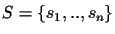is the order of the sequences that is derived from the optimal solution of a TSP, where the distances between the sequences are the pairwise PAM distances.

Definition 2.3   A tour Ci is shorter than a tour Cj (Ci < Cj) if the number of edges that are traversed by Ci is larger than the number of edges that are traversed by Cj. If Ci < Cj then the score of the tour Ci is smaller than the score of the tour Cj (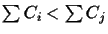).

Problem 2.1 (TSP problem)   Given is a set of sequencesand the corresponding scores of the optimal pairwise alignments. The problem is to find the shortest tour where each sequence is visited once.

Since we always talk about the same input sequences S, we will use C instead of C(S) and T instead of T(S). Let Cmin be the circular tour that is derived with a TSP algorithm. Since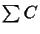is the score of an evolutionary tree,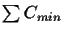is the minimum score.

Lemma 2.1   Cmin is a circular order of the optimal tree Tmin.

Proof 2.1   Assume Tmin does not have the circular order Cmin(S). We know that a circular order is the shortest tour through a tree, and any other ordering leads to a longer tour. Since Cmin does not belong to the set of circular orders of Tmin, then the tour that results from Cmin must be longer. Hence the score derived from Cmin cannot be optimal, which is a contradiction.

Lemma 2.2   There exists no tree with a lower score than Tmin.

Proof 2.2   Assume this statement is wrong and there exists a tree T' with a lower score than Tmin. Assume further that we know this tree T'. Hence we can derive a circular order C' easily. The sum of the PAM distances in this order (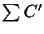) is the score of the tree. If T' really has a lower score, then the score derived from C' would have to be smaller than the score derived from Cmin, which is a contradiction.

In summary, we can compute a circular order Cmin without knowing T. With Cmin we can compute the minimum scoreof the unknown tree T.Next: Error Bound Up: Methods Previous: Scoring an Evolutionary Tree
Chantal Korostensky
1999-07-14